Motion Graphs and Forces Flashcards Preview

Physics > Motion Graphs and Forces > Flashcards

Flashcards in Motion Graphs and Forces Deck (105):
1

2

3

4

5

6

7

8

9

10

11

12

13

14

15

16

17

18

19

20

21

22

23

24

25

26

27

28

29

30

31

describe the motion of a on this distance/time graph32

describe the motion of e on this distance/time graph33

describe the motion of b and c on this distance/time graph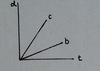34

describe the motion of f on this distance/time graph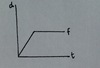35

describe the motion of a on this velocty/time graph36

describe the motion of d on this velocity/time graph37

describe the motion of b and c on this velocity/time graph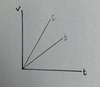38

39

calculate the resultant force and the accelertaion of this 5.0kg mass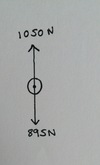40

calculate the resultant force and the accelertaion of this 5.0kg mass41

calculate the resultant force and the accelertaion of this 5.0kg mass42

calculate the resultant force and the accelertaion of this 5.0kg mass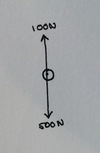43

what is the reaction time of the driver?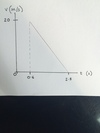44

calculate the thinking distance45

how long did the car brake for?46

calculate the braking distance47

calculate the stopping distance if it has been previously worked out that the thinking distance is 12m and the braking distance is 22m48

49

50

51

complete this skydiver velocity/time graph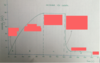52

state the value or direction of the resultant force at A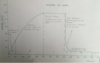53

state the value or direction of the resultant force at B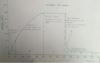54

state the value or direction of the resultant force at C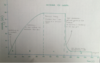55

state the value or direction of the resultant force at D56

57

58

59

60

61

which force is the foward force from the engine?which force is the force resisting the van's motion?62

complete this table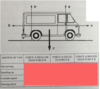63

64

65

66

67

68

69

70

what part of the graph represents thinking time of the driver and what is this time in seconds?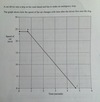71

calculate the distance travelled by the car in thinking time72

calculate the acceleration of the car after the brakes are applied73

calculate the distance travelled by the car during braking74

the mass of the car is 800kgcalculate the braking force75

76

77

78

79

80

81

82

83

84

85

86

87

88

89

90

91

92

What four forces are acting on a moving car?93

94

95

96

97

98

99

How can you investigate falling objects using sycamore seeds?

1. Sycamore seeds have a small wieght and a large surface area so they reach terminal velocity really quickly and fall slowly2. Collect a bunch of sycamore seeds of different sizes and measure the mass and wing length of each one. Use an accurate ruler and repeat each measurement several times to make sure it's accurate. Use all the seeds that have similar masses but different wavelengths3. Drop each of the seeds from the same height and use a stopwatch to find how long each one takes to fall to the ground. The higher you drop them the better - it gives a larger measurement and so improves the accuracy of the measurement4. Repeat the experiment for each seed and find an average time5. Plot a graph of length of the wings (x) against the time taken to hit the floor (y). This will tell you if there is a relationship between the shape of the sycamore seeds and their terminal velocity6. Bigger wings means bigger surface area and so higher drag. Higher drag means lower terminal velcoty, and so the seeds fall more slowly100

101

102

103

Be able to describe the motion of objects on a distance/time graph104

Be able to describe the motion of objects on a velocity/time graph105